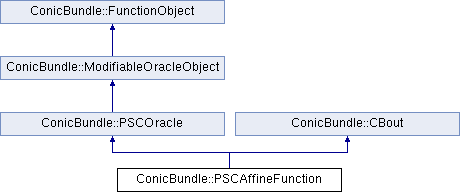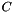ConicBundle
ConicBundle::PSCAffineFunction Class Reference

general purpose implementation of PSCOracle as explained in implemention of a PSCOracle (PSCAffineFunction) More...

`#include <PSCAffineFunction.hxx>`

Inheritance diagram for ConicBundle::PSCAffineFunction:## Public Member Functions

Initialization and setting parameters
PSCAffineFunction (const CBout *cb=0, int incr=-1)

PSCAffineFunction (const SparseCoeffmatMatrix &C, const SparseCoeffmatMatrix &opAt, PSCPrimal *generating_primal=0, const CBout *cb=0, int incr=-1)
initialize the PSCAffineFunction with its matrices and possible a generating_primal More...

~PSCAffineFunction ()

void set_check_correctness (bool chk)
set the maximum number of Ritz vectors returned in evaluate(), see implemention of a PSCOracle (PSCAffineFunction) More...

void set_max_Ritzvecs (CH_Matrix_Classes::Integer maxv)
set the maximum number of new Ritzvectors returned by evaluate(); values<1 default to 5

Implementations of PSCOracle routines
Minorantgenerate_minorant (const CH_Matrix_Classes::Matrix &P)
see PSCOracle::generate_minorant()

int svec_projection (CH_Matrix_Classes::Matrix &svec_offset, CH_Matrix_Classes::Matrix &svec_coeffs, const CH_Matrix_Classes::Matrix &P, const CH_Matrix_Classes::Indexmatrix *index_subset=0)
see PSCOracle::svec_projection()

int evaluate (const CH_Matrix_Classes::Matrix &current_point, const CH_Matrix_Classes::Matrix &bundlevecs, const double relprec, const double Ritz_bound, CH_Matrix_Classes::Matrix &Ritz_vectors, CH_Matrix_Classes::Matrix &Ritz_values, PSCPrimalExtender *&primal_extender)
see PSCOracle::evaluate()

int evaluate_projection (const CH_Matrix_Classes::Matrix &current_point, const CH_Matrix_Classes::Matrix &P, const double relprec, CH_Matrix_Classes::Matrix &projected_Ritz_vectors, CH_Matrix_Classes::Matrix &projected_Ritz_values)
see PSCOracle::evaluate_projection()

int left_right_product (int i, const CH_Matrix_Classes::Matrix &E, const CH_Matrix_Classes::Matrix &F, CH_Matrix_Classes::Matrix &G)
see PSCOracle::left_right_product()

virtual int apply_modification (const OracleModification &oracle_modification, const CH_Matrix_Classes::Matrix *new_center, const CH_Matrix_Classes::Matrix *old_center, bool &discard_objective_in_center, bool &discard_model, bool &discard_aggregates, MinorantExtender *&minorant_extender)
see PSCOracle::apply_modification() for the general use, here oracle_modification has a special role if it can be cast to an PSCAffineModification More...

virtual bool check_correctness () const
see PSCOracle::check_correctness()

routines for querying data of the problem
const SparseCoeffmatMatrixget_opAt ()

const SparseCoeffmatMatrixget_C ()

const PSCPrimalget_generating_primal (void)
returns the current setting concerning the generation of an PSCPrimal (0 for none)

routines for supporting input and output
void set_out (std::ostream *o=0, int pril=1)
see ConicBundle::CBout

void set_cbout (const CBout *cb, int incr=-1)
see ConicBundle::CBout

std::ostream & print_problem_data (std::ostream &out) const
write the problem description to out so that it can be read again by read_problem_data()

clear() and read the problem from in in the format written by print_problem_data()

std::ostream & print_problem_data_to_mfile (std::ostream &out, CH_Matrix_Classes::Integer blocknr) const
undocumented highly volatile variant for external testingPublic Member Functions inherited from ConicBundle::ModifiableOracleObject
virtual ~ModifiableOracleObject ()
virtual destructorPublic Member Functions inherited from ConicBundle::CBout
void clear_cbout ()
reset to default settings (out=0,print_level=1)

CBout (const CBout *cb=0, int incr=-1)
calls set_cbout

CBout (std::ostream *outp, int pl=1)
initialize correspondingly

CBout (const CBout &cb, int incr=0)
copy constructor

virtual bool cb_out (int pl=-1) const
Returns true if out!=0 and (pl<print_level), pl<0 should be used for WARNINGS and ERRORS only, pl==0 for usual output.

std::ostream & get_out () const
If cb_out() returned true, this returns the output stream, but it will abort if called with out==0.

std::ostream * get_out_ptr () const
returns the pointer to the output stream

int get_print_level () const
returns the print_level

virtual int mfile_data (std::ostream &out) const
writes problem data to the given outstream

## Private Member Functions

int form_bigmatrix (const CH_Matrix_Classes::Matrix &current_point)
compute the Bigmatrix representation for the given point

int apply_modification (const PSCAffineModification &amfmod)
applies the PSCAffineModfication amfmod to the current function

void clear ()
resets all to the initial empty state

## Private Attributes

SparseCoeffmatMatrix C
block diagonal representation ofas in implemention of a PSCOracle (PSCAffineFunction)

SparseCoeffmatMatrix opAt
holds the coefficient matrices for the variables

PSCPrimalgenerating_primal
This PSCPrimal can be set from outside and serves for generating further primals by cloning.

std::vector< AMFMaxEigSolver * > maxeigsolver
the eigenvalue solver of each block

CH_Matrix_Classes::Matrix last_bigmat_y
the last y used in costructing the matrix of the affine matrix function

CH_Matrix_Classes::Integer maxvecs
the maximum number of Ritz vectors to be retrurned

bool check_correctness_flag
if true, ConicBundle employs some additional consistency checks

## Detailed Description

general purpose implementation of PSCOracle as explained in implemention of a PSCOracle (PSCAffineFunction)

The documentation for this class was generated from the following file: